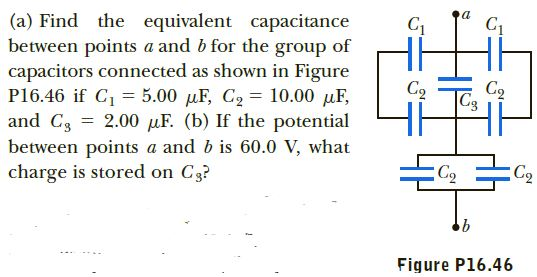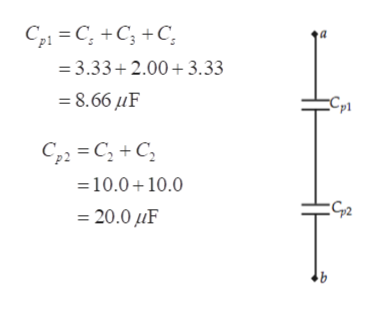# Hi(a) Find the equivalent capacitancebetween points a and b for the group ofcapacitors connected as shown in FigureP16.46 if C, = 5.00 µF, C2 = 10.00 µF,and C3 = 2.00 µF. (b) If the potentialbetween points a and b is 60.0 V, whatcharge is stored on C3?Cq|C3C2Figure P16.46

Question
4 viewshelp_outlineImage TranscriptioncloseHi (a) Find the equivalent capacitance between points a and b for the group of capacitors connected as shown in Figure P16.46 if C, = 5.00 µF, C2 = 10.00 µF, and C3 = 2.00 µF. (b) If the potential between points a and b is 60.0 V, what charge is stored on C3? Cq |C3 C2 Figure P16.46 fullscreen
check_circle

Step 1

Step 1

The top two capacitors are in series (on both sides are the same), the equivalent capacitance is C

Step 2

Step 2

Now, combine the top three that are in parallel

Then combine the bottom two that are in parallel, to gethelp_outlineImage TranscriptioncloseC21 = C; +C; +C, = 3.33+ 2.00 +3.33 = 8.66 µF Cp1 C22 = C, + C, =10.0+ 10.0 EG2 = 20.0 µF 91 fullscreen
Step 3

Step 3

Combining the remaining two ...

### Want to see the full answer?

See Solution

#### Want to see this answer and more?

Solutions are written by subject experts who are available 24/7. Questions are typically answered within 1 hour.*

See Solution
*Response times may vary by subject and question.
Tagged in

### Current Electricity